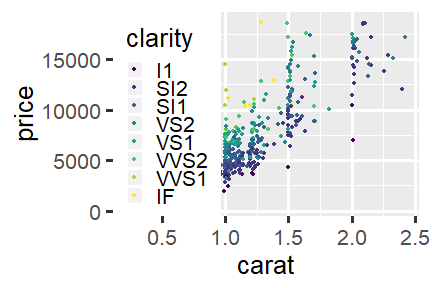## Legend functions

• Extract legend: `g_legend`
• Reposition legend onto the actual plot: `reposition_legend`
• Combine multiple plots, but use only one legend: `grid_arrange_shared_legend`

## Reposition legend onto plotting panel

`ggplot2` by default places the legend in the margin of the entire plot. This is in many instances a nice solution. If this is not desired, `theme(legend.position)` can be used to place the legend in relative measures on the entire plot:

``````library(ggplot2)
library(grid)
library(gridExtra)
dsamp <- diamonds[sample(nrow(diamonds), 1000), ]
(d <- ggplot(dsamp, aes(carat, price)) +
geom_point(aes(colour = clarity)) +
theme(legend.position = c(0.06, 0.75))
)
``````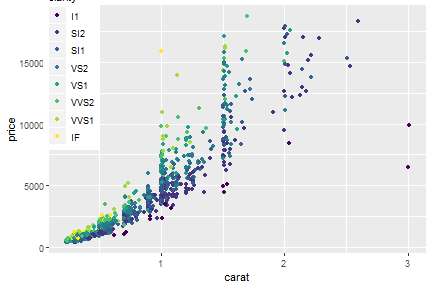This is however prone to badly positioning, if e.g. the plot is resized or font size changed: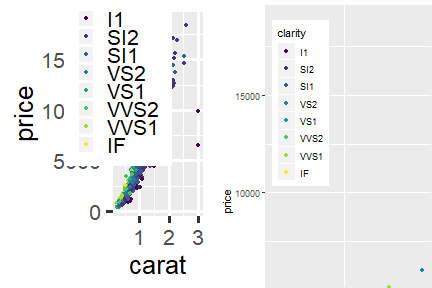With our function, we can specify exactly how we want it in the plotting area:

``````library(lemon)
reposition_legend(d, 'top left')
``````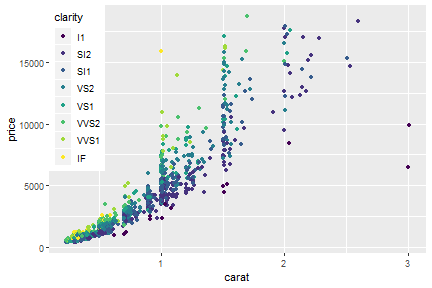And it stays there.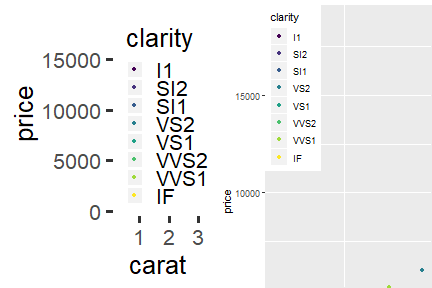The left plot is printed in full size at the end of this document.

### Multiple legends per guide

For our final trick in this act, we reposition a legend with multiple guides. For this, use `theme(legend.box.background)` to put a background around the entire legend, not just the individual guides.

``````d2 <- d + aes(shape=cut) +
theme(legend.box.background = element_rect(fill='#fffafa'),
legend.background = element_blank())
reposition_legend(d2, 'left')
``````
``````## Warning: Using shapes for an ordinal variable is not advised

## Warning: Using shapes for an ordinal variable is not advised
``````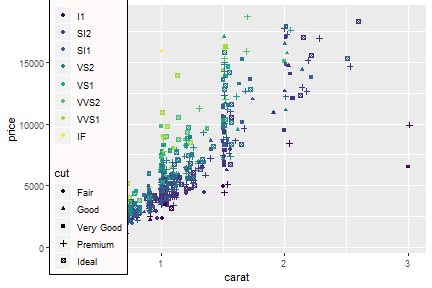### Legends are placed under axis lines

The guidebox uses a solid background (subject to the chosen theme), and prior to lemon version 0.3.1, the entire legend was placed as the top most element. In the examples above, this was not an issue. With axis lines drawn, this effectively overpainted some of the axis (same applies to the panel border).

The guidebox is therefore placed under the lowest axis line, if and only if `z = Inf`. To place as top most, specify a large z-index.

``````reposition_legend(d + theme_classic(), 'top left')
``````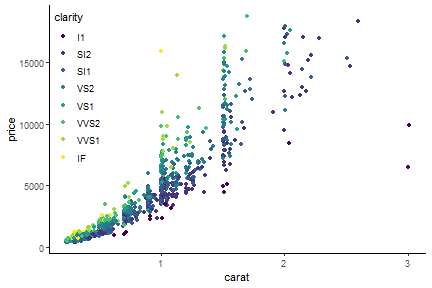To adjust the guidebox so it does not overpaint the panel border, use arguments `x` and `y`,

``````reposition_legend(d + theme_bw(), 'top left', x=0.002, y=1-0.002)
``````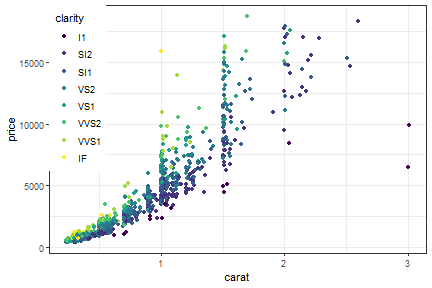… or use the argument `offset`:

``````reposition_legend(d + theme_bw(), 'top left', offset=0.002)
``````### Warning regarding extracting legend

To our knowledge, there exists two methods for extracting the legend:

``````g1 <- function(a.gplot){
if (!gtable::is.gtable(a.gplot))
a.gplot <- ggplotGrob(a.gplot)
leg <- which(sapply(a.gplot\$grobs, function(x) x\$name) == "guide-box")
a.gplot\$grobs[[leg]]
}
g2 <- function(a.gplot){
if (!gtable::is.gtable(a.gplot))
a.gplot <- ggplotGrob(a.gplot)
gtable::gtable_filter(a.gplot, 'guide-box', fixed=TRUE)
}
``````

There is very little difference between them, as the latter essentially does the same as the former. The latter however encapsulated the former in a gtable. This is even more evident with multiple guides:

``````(da <- ggplot(dsamp, aes(carat, price)) +
geom_point(aes(colour = clarity, shape=cut)) +
theme(legend.box = 'horizontal')
)
``````
``````## Warning: Using shapes for an ordinal variable is not advised
``````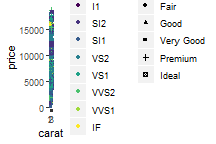``````print(g1(da))
``````
``````## Warning: Using shapes for an ordinal variable is not advised
``````
``````## TableGrob (5 x 7) "guide-box": 3 grobs
##                                     z     cells                  name
## 99_86c3b14d399915779b8ee8f468a98f91 1 (3-3,3-3)                guides
## 99_e48d95bbf9bfaae50e1c3ba1663f2112 2 (3-3,5-5)                guides
##                                     0 (2-4,2-6) legend.box.background
##                                               grob
## 99_86c3b14d399915779b8ee8f468a98f91 gtable[layout]
## 99_e48d95bbf9bfaae50e1c3ba1663f2112 gtable[layout]
##                                     zeroGrob[NULL]
``````
``````print(g2(da))
``````
``````## Warning: Using shapes for an ordinal variable is not advised
``````
``````## TableGrob (1 x 1) "layout": 1 grobs
##    z     cells      name              grob
## 1 14 (1-1,1-1) guide-box gtable[guide-box]
``````

The function `reposition_legend` assumes the method given in `g1`, which is also given in `g_legend`.

### Placing the legend in facets

The above demonstration finds the panel named `panel`. This is default. If using facetting, the panels are typically named `panel-{column}-{row}`. We use `gtable_show_names` to display the names of the facetted panels.

``````d2 <- d + facet_grid(.~cut)
gtable_show_names(d2)
``````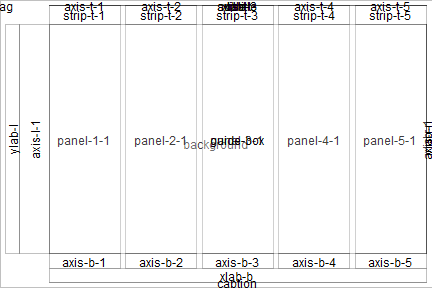So to place the legend in a specific panel, give its name:

``````reposition_legend(d2, 'top left', panel = 'panel-3-1')
``````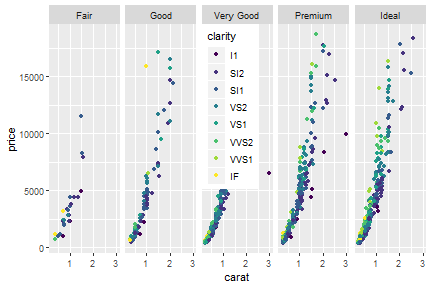Likewise for `facet_wrap`. Incidentally, empty panels are also named here:

``````reposition_legend(d + facet_wrap(~cut, ncol=3), 'top left', panel='panel-3-2')
``````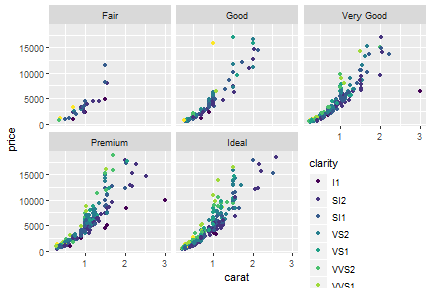Modifying the legend is done via usual routines of ggplot2:

``````d3 <- d + facet_wrap(~cut, ncol=3) + scale_color_discrete(guide=guide_legend(ncol=3))
reposition_legend(d3, 'center', panel='panel-3-2')
``````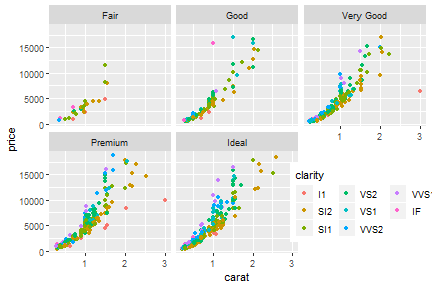Also supports spanning multiple panels:

``````d4 <- d + facet_wrap(~cut, ncol=4) + scale_color_discrete(guide=guide_legend(nrow=2))
reposition_legend(d4, 'center', panel=c('panel-2-2','panel-4-2'))
``````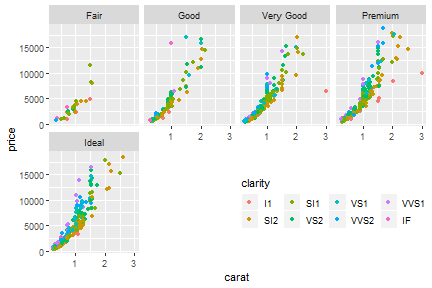The panel names are not easy to figure, especially those from `facet_wrap`. We refer to `gtable_show_names` to get a look at where they are:

``````gtable_show_names(d4)
``````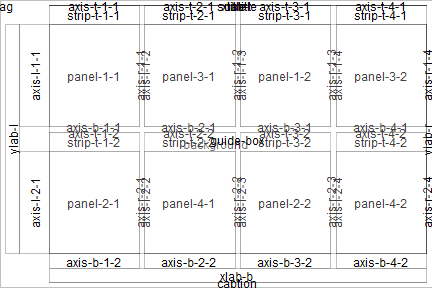## Shared legend across multiple plot

The function `grid_arrange_shared_legend` extracts the legend from its first argument, combines the plots with the legend hidden using `arrangeGrob`, and finally appends the legend to one of the sides. It even updates the plot's theme to orientate the legend correctly.

``````dsamp <- diamonds[sample(nrow(diamonds), 1000), ]
p1 <- qplot(carat, price, data = dsamp, colour = clarity)
p2 <- qplot(cut, price, data = dsamp, colour = clarity)
p3 <- qplot(color, price, data = dsamp, colour = clarity)
p4 <- qplot(depth, price, data = dsamp, colour = clarity)
grid_arrange_shared_legend(p1, p2, p3, p4, ncol = 2, nrow = 2, position='top')
``````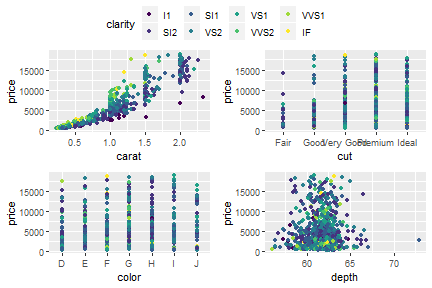``````grid_arrange_shared_legend(p1, p2, p3, p4, ncol = 2, nrow = 2, position='bottom')
``````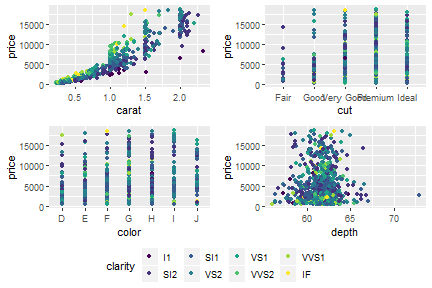``````grid_arrange_shared_legend(p1, p2, p3, p4, ncol = 2, nrow = 2, position='left')
``````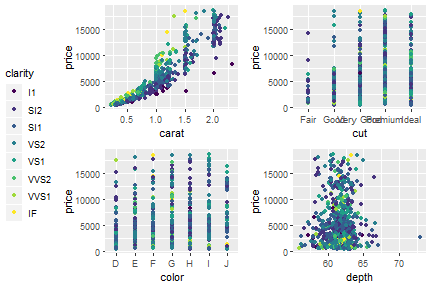``````grid_arrange_shared_legend(p1, p2, p3, p4, ncol = 2, nrow = 2, position='right')
``````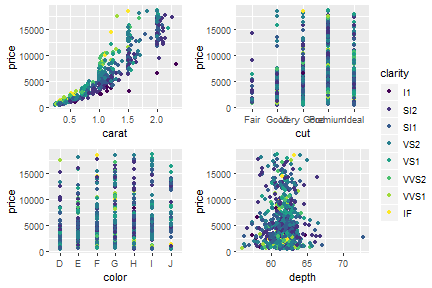## Shared legend with `grid.arrange`

A more flexible approach to combining plots and legends can be found in Baptiste Auguie's `gridExtra`::`grid.arrange` and `arrangeGrob`. The latter is the power house that produces a grob object, which the former then draws to the device. But being more flexible, it is somewhat less automated.

We demonstrate here how to combine 3 of the 4 plots above, with different options for layout and placing the legend.

``````library(gridExtra)
legend <- g_legend(p1 + theme(legend.position='bottom'))
grid.arrange(p1+theme(legend.position='hidden'), p2+theme(legend.position='hidden'),
p3+theme(legend.position='hidden'), legend)
``````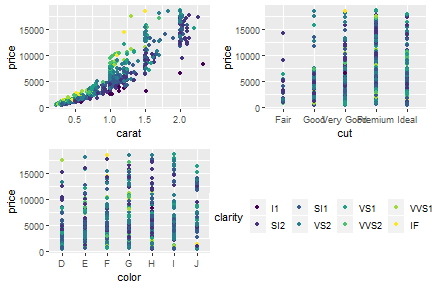``````grid.arrange(p1+theme(legend.position='hidden'), p2+theme(legend.position='hidden'),
p3+theme(legend.position='hidden'), legend,
layout_matrix=matrix(c(1,3,4,2,3,4), ncol=2))
``````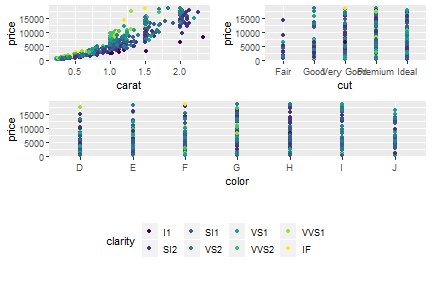In the figure above, a layout matrix was defined with three rows. Subsequently, the three rows were of equal heights.

It is difficult to calculate the row heights to provide `grid.arrange` if we wanted to fix the height of the legend row's height. We could attempt

``````(unit(1, 'npc') - sum(legend\$heights)) / 2
``````

but division is not permitted on units.

When `grid.arrange` is given a margin argument (e.g. `bottom`), it creates a gtable object with a row or column of appropiate dimension. The remainder of the gtable is re-sized to fit. We can therefore do:

``````grid.arrange(p1+theme(legend.position='hidden'), p2+theme(legend.position='hidden'),
p3+theme(legend.position='hidden'), bottom=legend\$grobs[],
layout_matrix=matrix(c(1,3,2,3), ncol=2))
``````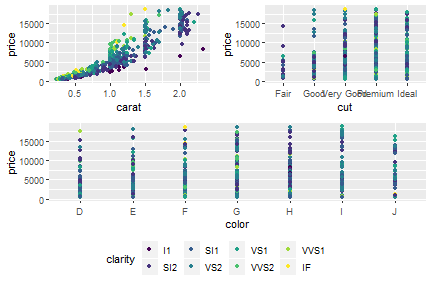There is however currently a shortcoming in `grid.arrange` and `arrangeGrob` that prevents it from using the full gtable object returned from `g_legend` in the margin arguments. We therefore subset to a specific grob.

What worse is, if the legend contains multiple guides, the above approach will not work directly. However, defining several `arrangeGrob` within each other is possible.

## More examples

### Complex layout with grid_arrange_shared_legend

In this reproducible example grid plot, 3 plots have 3 fill colours, and z displays with the “col” blue, but in the fourth plot there is only 1 “col”, so z displays as red.

I want to show only one common legend (which I can do), but I want z to be blue in all four plots. Is there a simple way to do that?

Before:

``````library(ggplot2)
library(grid)
library(gridExtra)

p0 <- ggplot(d0) + geom_col(mapping = aes(x, y, fill = col))
p1 <- ggplot(d1) + geom_col(mapping = aes(x, y, fill = col))
p2 <- ggplot(d2) + geom_col(mapping = aes(x, y, fill = col))
p3 <- ggplot(d3) + geom_col(mapping = aes(x, y, fill = col))
grid.arrange(p0, arrangeGrob(p1,p2,p3, ncol=3), ncol=1)
``````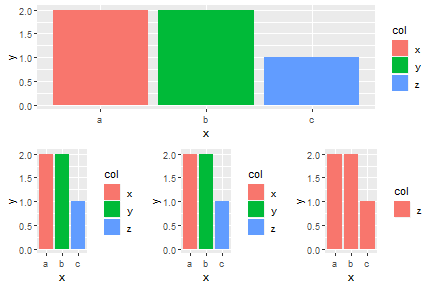``````nt <- theme(legend.position='hidden')
grid_arrange_shared_legend(p0, arrangeGrob(p1+nt,p2+nt,p3+nt, ncol=3), ncol=1, nrow=2)
``````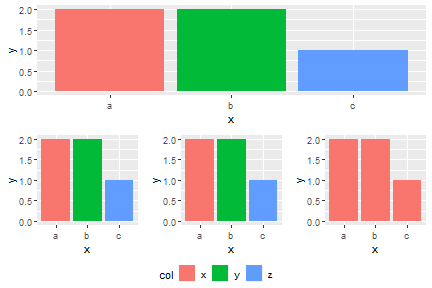## Plot for ggplot2-extensions

``````p <- ggplot(dsamp, aes(x=cut, y=price, colour=clarity)) + geom_point(position=position_jitter(width=0.2)) +
coord_flex_cart(bottom=brackets_horizontal(), left=capped_vertical('none')) +
theme_bw() + theme(panel.border=element_blank(), axis.line = element_line(),
legend.background = element_rect(colour='grey'))
g <- reposition_legend(p, 'top left', plot=TRUE)
``````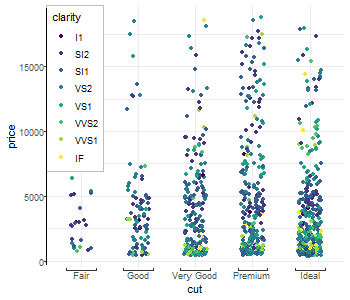## Acknowledgements

`g_legend` was proposed as early as June 2012 by Baptiste Auguié (http://baptiste.github.io/) on ggplot2's wiki. It has since propogated throughout Stack Overflow answers.

Originally brought to you by (Baptiste Auguié)http://baptiste.github.io/ and (Shaun Jackman)http://rpubs.com/sjackman. It has been further modified here.

`reposition_legend` was coded by Stefan McKinnon Edwards/

## Footnotes

Example with `reposition_legend` that didn't quite work:

``````dsamp <- diamonds[sample(nrow(diamonds), 1000), ]
d <- ggplot(dsamp, aes(carat, price)) +
geom_point(aes(colour = clarity))
reposition_legend(d + theme_gray(base_size=26), 'top left')
``````